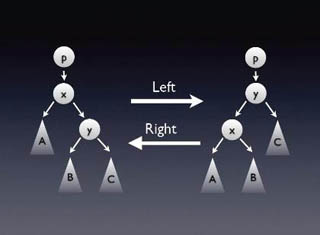6.006 | Spring 2008 | Undergraduate
Introduction to Algorithms
Course Description
This course provides an introduction to mathematical modeling of computational problems. It covers the common algorithms, algorithmic paradigms, and data structures used to solve these problems. The course emphasizes the relationship between algorithms and programming, and introduces basic performance measures and …
Course Info
Instructors
Departments
Topics
Learning Resource Types
assignment Problem Sets